## Suppose f(x) = x2 and g(x) = Which statement best compares the graph of g(x) with the graph of f(x)?​

Question

Suppose f(x) = x2 and g(x) = Which statement best compares the graph of g(x) with the graph of f(x)?​

in progress 0
3 weeks 2021-08-31T12:58:52+00:00 1 Answers 0 views 0

g(x) is horizontally stretched by a factor of 1/5

Step-by-step explanation:

Given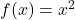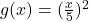Require

Determine the relationship between f(x) and g(x)Express g(x) in terms of f(x)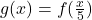This can further be simplified as: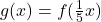When a function is represented as: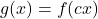Where c < 1,

Then the type of translation is a horizontal stretch (stretch in the x direction)

By comparison:and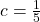This means that: g(x) is horizontally stretched by a factor of 1/5